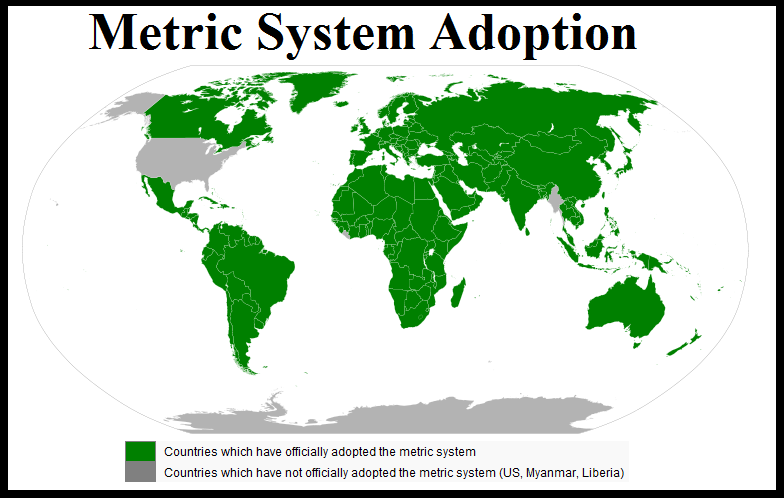### Strutt Help

Calculation Units

Strutt works in either Metric or Imperial (English Customary) units. Technically, all calculations in Strutt are undertaken in Metric units, with a series of Imperial-to-Metric conversion factors applied to the input data when Imperial units are selected. The selection of units is not retrospective, and will not affect any previously undertaken calculations on a worksheet. The units selection is only applied to calculations created after the selection of the input units.

The default for any new calculation in Strutt is metric units. Support for imperial units is added progressively. For context behind this decision, refer below:The units can be selected in the Strutt Preferences under Strutt|Settings|Preferences

Strutt implements the following conversion factors;

Inches to Metres

• Length = 39.37
• Area = 1550.003
• Volume = 61023.74
Inches to Millimetres
• Length = 1 / 25.4
Feet to Metres
• Length = 3.28
• Area = 10.76
• Volume = 35.31
Cubic Feet per minute (CFM) to m3/s
• Flowrate = 2118.88
Inches Water to Pascal
• Pressure = 1 / 249
Horsepower to kW
• Horsepower=1.341022
• Boiler Horsepower=0.101946
Tons cooling to kW
• Tons=0.284598
Surface Mass in lb/ft˛ to kg/m˛
• Surface Mass=4.882404